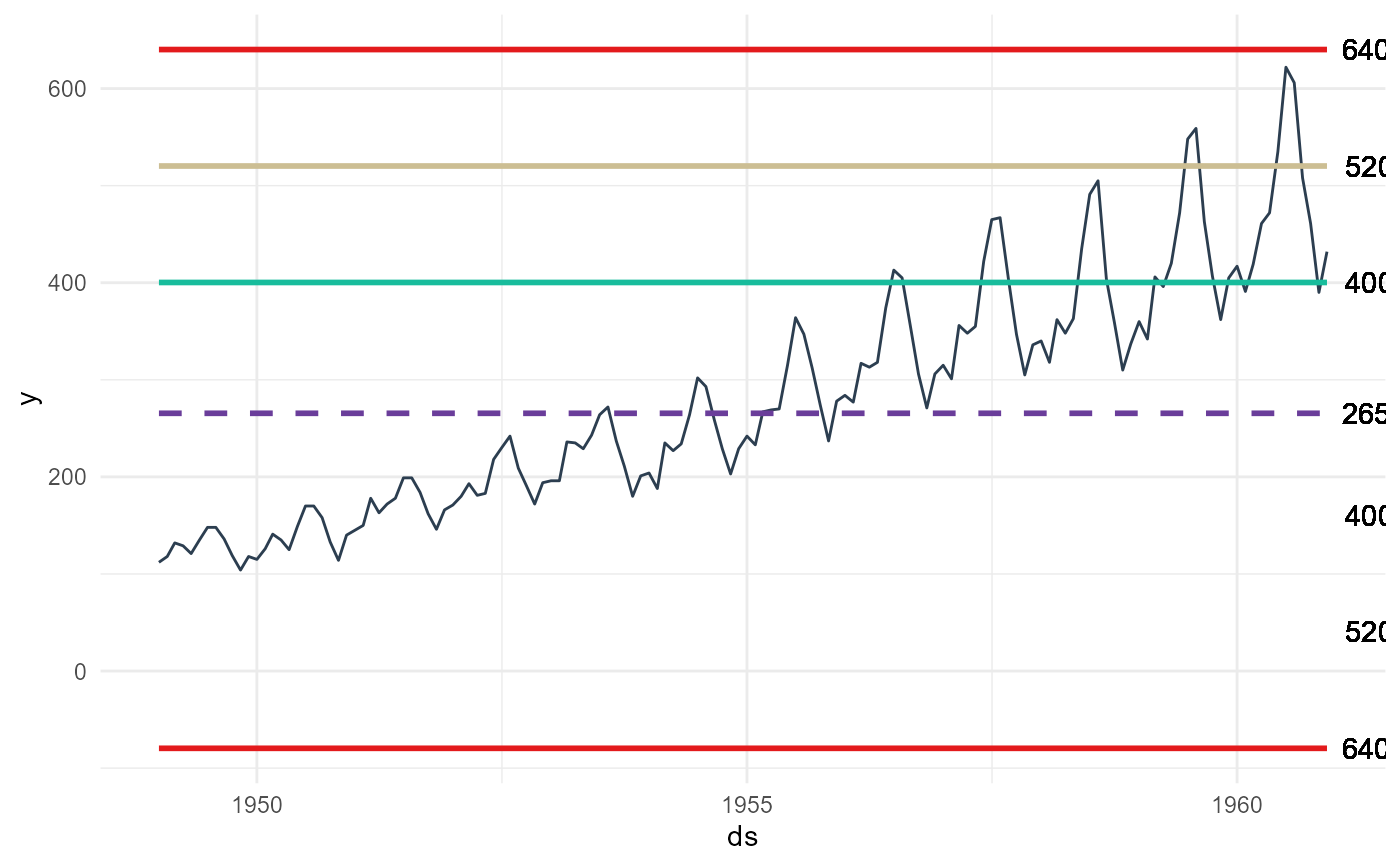A control chart is a specific type of graph that shows data points between upper and lower limits over a period of time. You can use it to understand if the process is in control or not. These charts commonly have three types of lines such as upper and lower specification limits, upper and lower limits and planned value. By the help of these lines, Control Charts show the process behavior over time.

## Usage

ts_qc_run_chart(
.data,
.date_col,
.value_col,
.interactive = FALSE,
.median = TRUE,
.cl = TRUE,
.mcl = TRUE,
.ucl = TRUE,
.lc = FALSE,
.lmcl = FALSE,
.llcl = FALSE
)

## Arguments

.data

The data.frame/tibble to be passed.

.date_col

The column holding the timestamp.

.value_col

The column with the values to be analyzed.

.interactive

Default is FALSE, TRUE for an interactive plotly plot.

.median

Default is TRUE. This will show the median line of the data.

.cl

This is the first upper control line

.mcl

This is the second sigma control line positive

.ucl

This is the third sigma control line positive

.lc

This is the first negative control line

.lmcl

This is the second sigma negative control line

.llcl

This si the thrid sigma negative control line

## Value

A static ggplot2 graph or if .interactive is set to TRUE a plotly plot

## Details

• Expects a time-series tibble/data.frame

• Expects a date column and a value column

## Author

Steven P. Sanderson II, MPH

## Examples

library(dplyr)

data_tbl <- ts_to_tbl(AirPassengers) %>%
select(-index)

data_tbl %>%
ts_qc_run_chart(
.date_col    = date_col
, .value_col = value
, .llcl      = TRUE
)